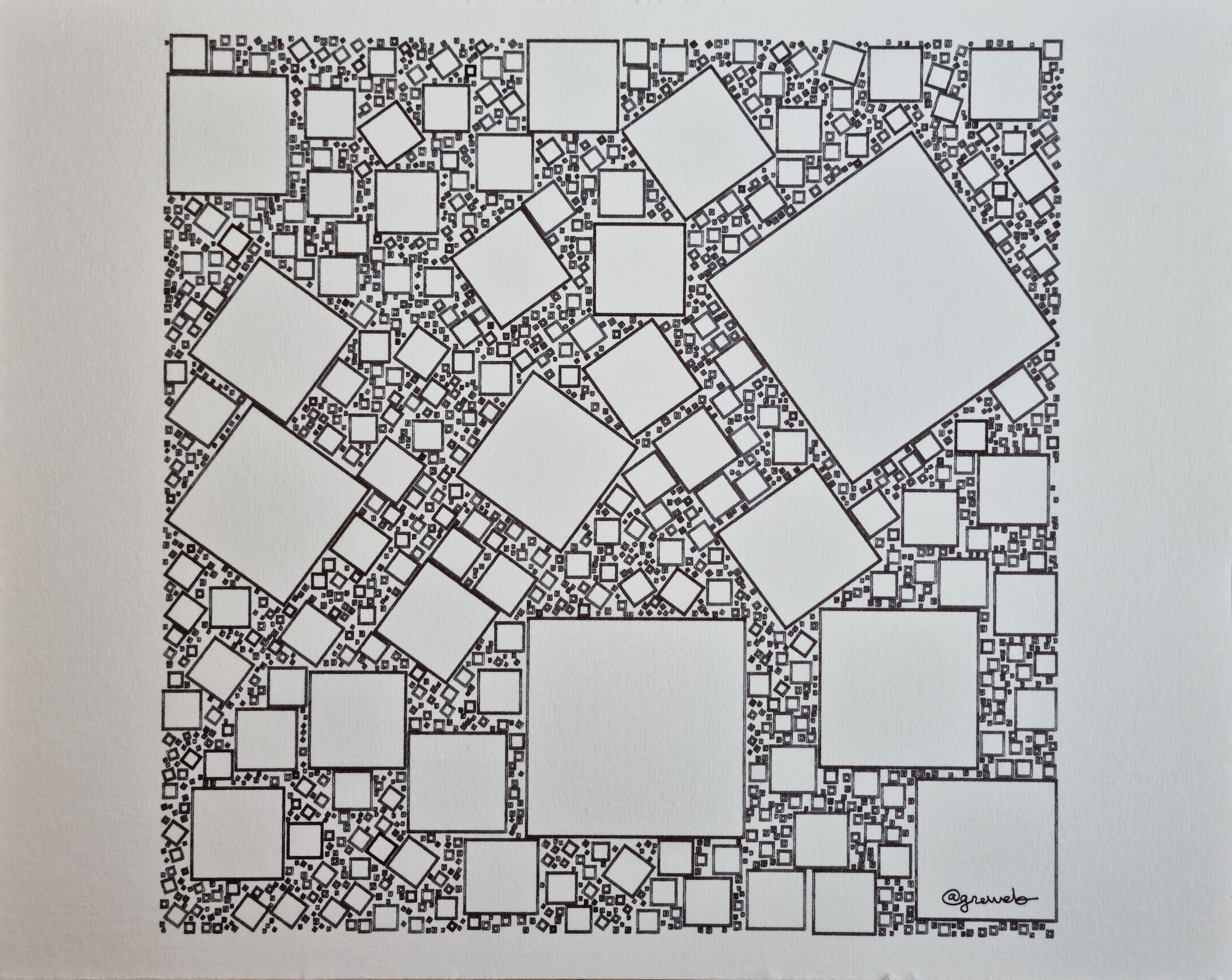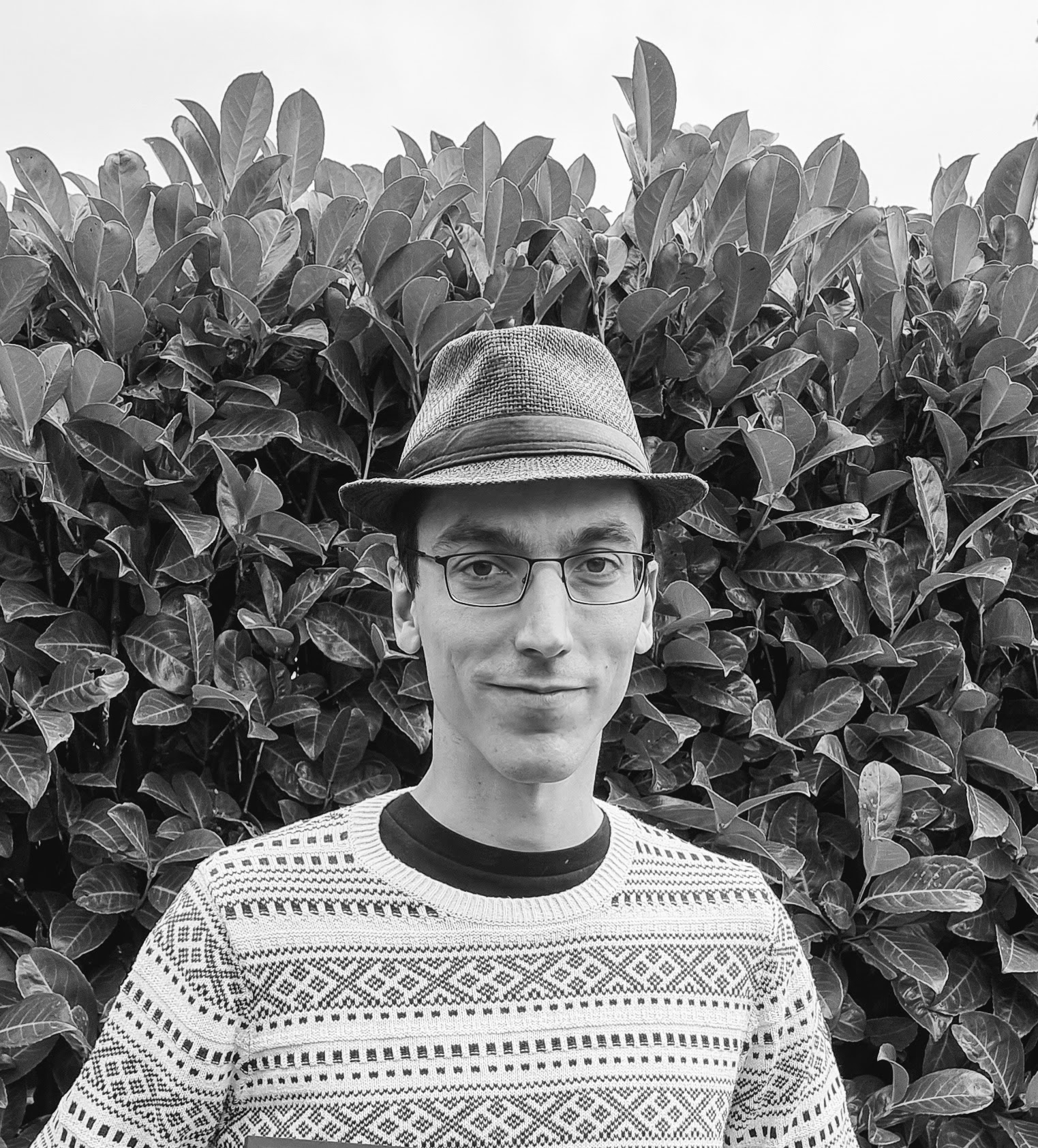# greweb.me / Plot #180 "squares packing 002"Continuation of plot#179.

Second iteration on packing rotated squares in a square. Black fountain pens on a 300g/m paper. It's interesting how the paper makes the ink having different shade of greys.

This time, the rotations are less random and aligned on 10 possible angles. (but these are squares so not it repeats)

There is also two concentric squares drawn each time.

## Technical notes

This is also a continuation of the code of plot#179.

I did an improvement of the search algorithm using a dichotomic search:

search

``````fn poly_square_scaling_search(
boundaries: (f64, f64, f64, f64),
polys: &Vec<Polygon<f64>>,
x: f64,
y: f64,
angle: f64,
min_scale: f64,
max_scale: f64,
) -> Option<f64> {
let overlaps = |size| {
let poly = rotated_square_as_polygon(x, y, size, angle);
let bounds = poly.bounding_rect().unwrap();
let topleft: Point<f64> = bounds.min().into();
let bottomright: Point<f64> = topleft + point!(
x: bounds.width(),
y: bounds.height()
);
out_of_boundaries(topleft.x_y(), boundaries)
|| out_of_boundaries(bottomright.x_y(), boundaries)
|| poly_collides_in_polys(polys, &poly)
};

let mut from = min_scale;
let mut to = max_scale;
loop {
if overlaps(from) {
return None;
}
if to - from < 0.1 {
return Some(from);
}
let middle = (to + from) / 2.0;
if overlaps(middle) {
to = middle;
}
else {
from = middle;
}
}
}
``````creative coder experimenting with GLSL shaders, Rust, and fountain pens robot plots. infinite noise explorer.